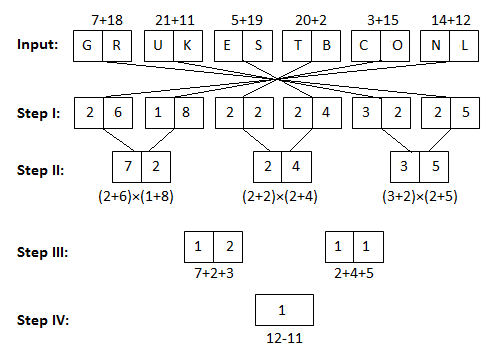# High Level Machine Input/output Quiz for IBPS PO Part-8

Directions (1 – 3): Study the following table carefully and answer the questions that follow:
The table shows the discount % given by stores on different items. The Market Price of any article on all stores is same. Some values are missing. Answer the questions on the basis of given table and information in question.

Article
Store 1
Store 2
Store 3
A
15%
8%
20%
B
27%
16%
-
C
16%
-
32%
D
-
-
20%
E
10%
12%
8%

### Question. 1.

If the average SP of article A by in all the stores is Rs 3598, Find the MP of article A.
A. 4500 Rs.
B. 3500 Rs.
C. 4000 Rs.
D. 4200 Rs.
E. None of these.
Ans. D.
Solution:### Question. 2.

Store 2 earned 10% profit by selling Article E. If CP of all articles is same for Article E, find the ratio of profits by Store 1 and 3 of Article E.
A. 2:3
B. 4:5
C. 4:3
D. 5:6
E. None of these.
Ans. D.
Solution: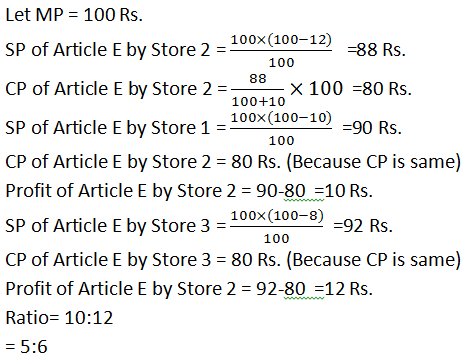### Question. 3.

Average SP of article B by stores 1 and 3 is Rs 3060, by stores 2 and 3 is Rs 3280. Find the SP of article B by store 3.
A. 30%
B. 20%
C. 25%
D. 15%
E. None of these.
Ans. B.
Solution: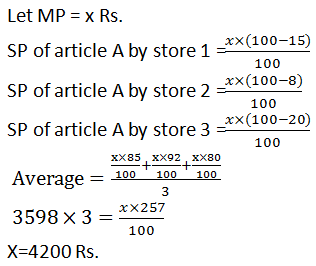Directions (Q. 4-7): Study the following information carefully and answer the questions given below:
A number or alphabet arrangement machine when given an input line of numbers or alphabets rearranges them following a particular rule in each step. The following is an illustration of input and various steps of rearrangement.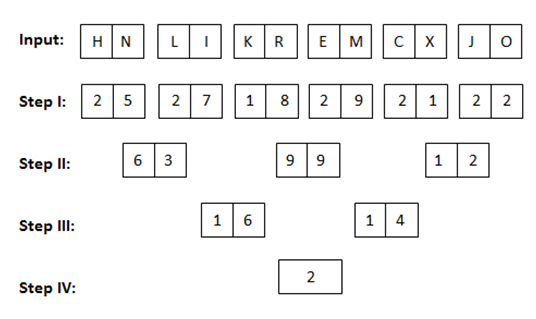And Step IV is the last step of the rearrangement as the desired arrangement is obtained. As per rules followed in the above steps, find out in each of the questions the appropriate step for the given input.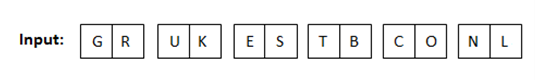### Question. 4.

Which of the following will be the last Step?
A. 5.3
B. 2
C. 1
D. 2.5
E. 3
Ans. C.
Solution:
Step IV: 1

### Question. 5.

If in the second step the second digit of the every number is added and multiply by 4 then which will be the resultant value?
A. 15
B. 44
C. 30
D. 25
E. 49
Ans. B.
Solution:
= (2+4+5)4
= 44

### Question. 6.

What is the sum of numbers in step III?
A. 35
B. 22
C. 14
D. 23
E. 29
Ans. D.
Solution:
=12+11
=23

### Question. 7.

Which of the following will be Step II?
A. 72, 24, 35
B. 65, 30, 42
C. 69, 85, 25
D. 55, 15, 35
E. None of these.
Ans. A.
Solution:
Step II: 72, 24, 35